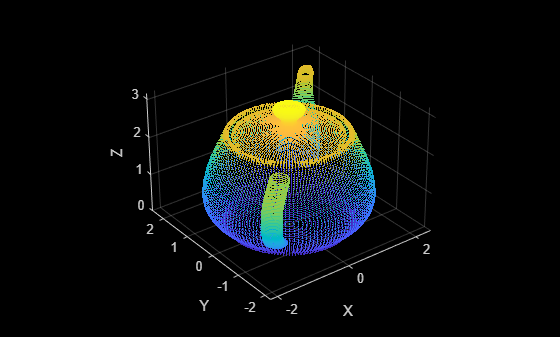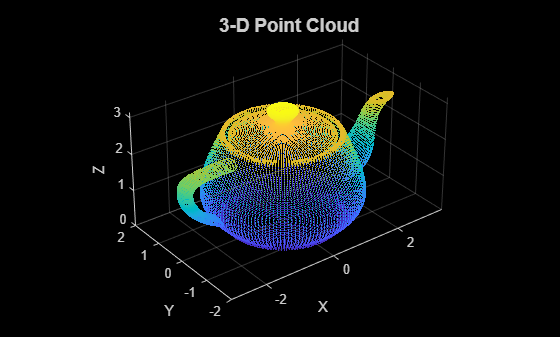# pctransform

Transform 3-D point cloud

## Syntax

``ptCloudOut = pctransform(ptCloudIn,tform)``
``ptCloudOut = pctransform(ptCloudIn,D)``

## Description

example

````ptCloudOut = pctransform(ptCloudIn,tform)` applies the specified 3-D affine transform, `tform` to the point cloud, `ptCloudIn`. The transformation can be a rigid or nonrigid transform. ```

example

````ptCloudOut = pctransform(ptCloudIn,D)` applies the displacement field `D` to the point cloud. Point cloud transformation using a displacement field define translations with respect to each point in the point cloud.```

## Examples

collapse all

`ptCloud = pcread('teapot.ply');`

Plot the point cloud.

```figure pcshow(ptCloud) xlabel('X') ylabel('Y') zlabel('Z')```Create a transform object with a 45-degree rotation along the Z-axis.

```theta = pi/4; rot = [cos(theta) sin(theta) 0; ... -sin(theta) cos(theta) 0; ... 0 0 1]; trans = [0, 0, 0]; tform = rigid3d(rot,trans);```

Transform the point cloud.

`ptCloudOut = pctransform(ptCloud,tform);`

Plot the transformed point cloud.

```figure pcshow(ptCloudOut) xlabel('X') ylabel('Y') zlabel('Z')```This example shows affine transformation of a 3-D point cloud. The specified forward transform can be a rigid or nonrigid transform. The transformations shown includes rotation (rigid transform) and shearing (nonrigid transform) of the input point cloud.

Read a point cloud into the workspace.

`ptCloud = pcread('teapot.ply');`

Rotation of 3-D Point Cloud

Create an affine transform object that defines a 45 degree rotation along the z-axis.

```A = [cos(pi/4) sin(pi/4) 0 0; ... -sin(pi/4) cos(pi/4) 0 0; ... 0 0 1 0; ... 0 0 0 1]; tform = affine3d(A);```

Transform the point cloud.

`ptCloudOut1 = pctransform(ptCloud,tform);`

Shearing of 3-D point cloud

Create an affine transform object that defines shearing along the x-axis.

```A = [1 0 0 0; ... 0.75 1 0 0; ... 0.75 0 1 0; ... 0 0 0 1]; tform = affine3d(A);```

Transform the point cloud.

`ptCloudOut2 = pctransform(ptCloud,tform);`

Display the Original and Affine Transformed 3-D Point Clouds

Plot the original 3-D point cloud.

```figure1 = figure('WindowState','normal'); axes1 = axes('Parent',figure1); pcshow(ptCloud,'Parent',axes1); xlabel('X'); ylabel('Y'); zlabel('Z'); title('3-D Point Cloud','FontSize',14)``````% Plot the rotation and shear affine transformed 3-D point clouds. figure2 = figure('WindowState','normal'); axes2 = axes('Parent',figure2); pcshow(ptCloudOut1,'Parent',axes2); xlabel('X'); ylabel('Y'); zlabel('Z'); title({'Rotation of 3-D Point Cloud'},'FontSize',14)``````figure3 = figure('WindowState','normal'); axes3 = axes('Parent',figure3); pcshow(ptCloudOut2,'Parent',axes3); xlabel('X'); ylabel('Y'); zlabel('Z'); title({'Shearing of 3-D Point Cloud'},'FontSize',14)```Read a point cloud into the workspace.

`ptCloud = pcread('teapot.ply');`

Create a displacement field `D` of same size as the point cloud.

`D = zeros(size(ptCloud.Location));`

Set the displacement field value along x-axis for the first half of the points to 7.

```pthalf = ptCloud.Count/2; D(1:pthalf,1) = 7;```

Extract the indices of points within a region-of-interest (ROI) using the `pointCloud` method `findNeighborsInRadius`. Set the displacement field value along the x-, y-, and z-axis for points within the ROI to 4, 4, and -2, respectively.

```indices = findNeighborsInRadius(ptCloud,[0 0 3.1],1.5); D(indices,1:2) = 4; D(indices,3) = -2;```

Transform the point cloud using the displacement field.

`ptCloudOut = pctransform(ptCloud,D);`

Display the original and transformed point cloud.

```figure pcshow(ptCloud) xlabel('X'); ylabel('Y'); zlabel('Z'); title('Original 3-D Point Cloud')``````figure pcshow(ptCloudOut) xlabel('X'); ylabel('Y'); zlabel('Z'); title('Transformed 3-D Point Cloud Using Displacement Field')```## Input Arguments

collapse all

Point cloud, specified as a `pointCloud` object.

3-D geometric transformation, specified as a `rigid3d` object or an `affine3d` object. See Define Transformation Matrix (Image Processing Toolbox) for details on how to set up an affine 3-D `tform` input.

Displacement field, specified as either M-by-3 or an M-by-N-by-3 array. The displacement field is a set of displacement vectors that specify the magnitude and direction of translation for each point in the point cloud. The size of the displacement field must be the same as the size of the `Location` property of the `pointCloud` object.

Data Types: `single` | `double`

## Output Arguments

collapse all

Transformed point cloud, returned as a `pointCloud` object. The transformation applies to the coordinates of points and their normal vectors.